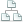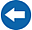Home >Tips >Excel 2016 from Scratch >Calculate time differencesSite map

# An Excel tutorial by Peter KalmstromIn this demo in the Excel 2016 from Scratch series, Peter Kalmstrom shows that what we have learned so far about numbers also is valid for times.

With train scheduled and actual arrivals as an example, Peter shows how to calculate the difference in time and then also the maximum, minimum and median differences.

When there are only positive values, which means that the train is never early, a simple calculation of the arrival time minus the scheduled time will give us the information we need.

However, a calculation of time never works with negative figures, so to cover all cases we need to format the cell contents as numbers and create a more advanced formula.

### Exercise

If you want to try the exercise yourself, please download the Excel file Peter uses in the demo.

### Content

This is what Peter shows in the demo below:
• How to see the difference between numerical cell content and other content.
• How to calculate the difference between two times.
• How to format cell content as numbers.
• How to create a formula that converts into minutes the decimal value you get from when calculating time.
• How to create a formula that finds the maximum value of the values in the selected cells.
• How to create a formula that finds the minimum value of the values in the selected cells.
• How to create a formula that calculates the median value from the values in the selected cells.

Peter uses Excel 2016 for his demo, but the Excel basics are the same for earlier versions of Excel.